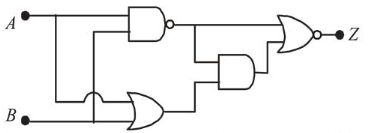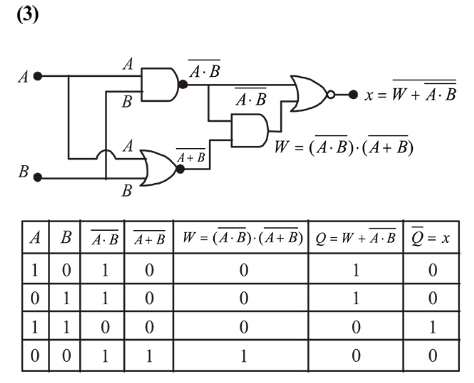# In the following digitial circuit, what will be the output at ' Z ',Question:

In the following digitial circuit, what will be the output at ' $Z$ ', when the input $(A, B)$ are $(1,0),(0,0),(1,1),(0,1)$ :1. $0,0,1,0$

2. $1,0,1,1$

3. $1,1,0,1$

4. $0,1,0,0$

Correct Option: , 3

Solution: